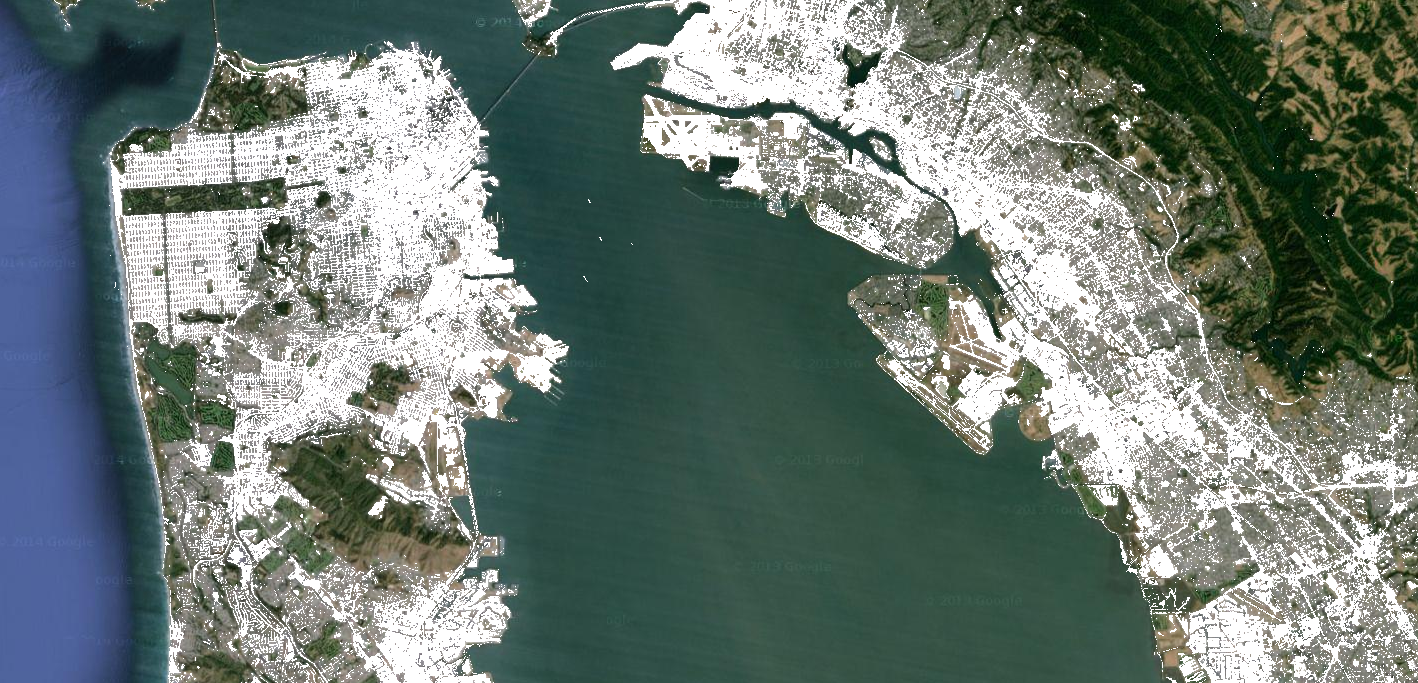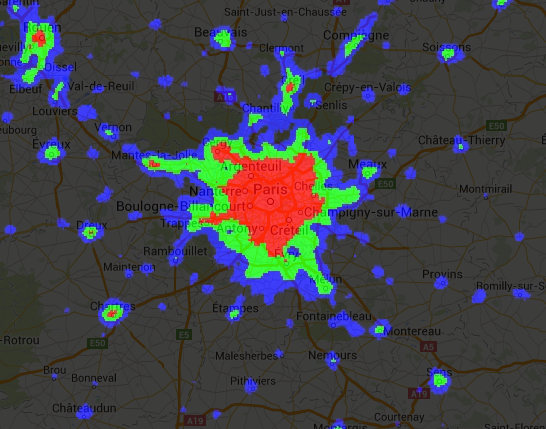# Relational, conditional and Boolean operations

To perform per-pixel comparisons between images, use relational operators. To extract urbanized areas in an image, this example uses relational operators to threshold spectral indices, combining the thresholds with `and()`:

```// Load a Landsat 8 image.
var image = ee.Image('LANDSAT/LC08/C01/T1_TOA/LC08_044034_20140318');

// Create NDVI and NDWI spectral indices.
var ndvi = image.normalizedDifference(['B5', 'B4']);
var ndwi = image.normalizedDifference(['B3', 'B5']);

// Create a binary layer using logical operations.
var bare = ndvi.lt(0.2).and(ndwi.lt(0));

// Mask and display the binary layer.
Map.setCenter(-122.3578, 37.7726, 12);
Map.setOptions('satellite');
```

As illustrated by this example, the output of relational and boolean operators is either true (1) or false (0). To mask the 0's, you can mask the resultant binary image with itself. The result from the previous example should look something like Figure 1.Figure 1. Low NDVI and low NDWI (white) from Landsat 8, San Francisco, California, USA.

The binary images that are returned by relational and boolean operators can be used with mathematical operators. This example creates zones of urbanization in a nighttime lights image using relational operators and `image.add()`:

```// Load a 2012 nightlights image.
var nl2012 = ee.Image('NOAA/DMSP-OLS/NIGHTTIME_LIGHTS/F182012');
var lights = nl2012.select('stable_lights');

// Define arbitrary thresholds on the 6-bit stable lights band.

// Display the thresholded image as three distinct zones near Paris.
var palette = ['000000', '0000FF', '00FF00', 'FF0000'];
Map.setCenter(2.373, 48.8683, 8);
Map.addLayer(zones, {min: 0, max: 3, palette: palette}, 'development zones');
```

Note that the code in the previous example is equivalent to using a ternary operator implemented by `expression()`:

```// Create zones using an expression, display.
var zonesExp = nl2012.expression(
"(b('stable_lights') > 62) ? 3" +
": (b('stable_lights') > 55) ? 2" +
": (b('stable_lights') > 30) ? 1" +
": 0"
);
{min: 0, max: 3, palette: palette},
'development zones (ternary)');
```

Observe that in the previous expression example, the band of interest is referenced using the `b()` function, rather than a dictionary of variable names. (Learn more about image expressions on this page. Using either mathematical operators or an expression, the output is the same and should look something like Figure 2.Figure 2. Arbitrary zones of 2012 nightlights imagery for Paris, France.

Another way to implement conditional operations on images is with the `image.where()` operator. Consider the need to replace masked pixels with some other data. In the following example, cloudy pixels are replaced by pixels from a cloud-free image using `where()`:

```// Load a cloudy Landsat 8 image.
var image = ee.Image('LANDSAT/LC08/C01/T1_TOA/LC08_044034_20130603');
{bands: ['B5', 'B4', 'B3'], min: 0, max: 0.5},
'original image');

// Load another image to replace the cloudy pixels.
var replacement = ee.Image('LANDSAT/LC08/C01/T1_TOA/LC08_044034_20130416');

// Compute a cloud score band.
var cloud = ee.Algorithms.Landsat.simpleCloudScore(image).select('cloud');

// Set cloudy pixels to the other image.
var replaced = image.where(cloud.gt(10), replacement);

// Display the result.
Map.centerObject(image, 9);
In this example, observe the use of the `simpleCloudScore()` algorithm. This algorithm ranks pixels by cloudiness on a scale of 0-100, with 100 most cloudy. Learn more about `simpleCloudScore()` on the Landsat Algorithms page.